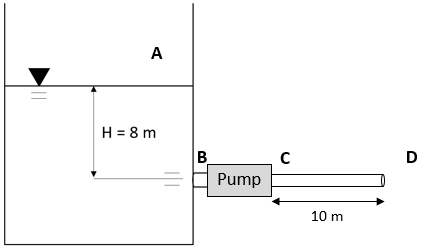## Fluid Flow

In the figure below, water is pumped through a 50 mm diameter pipe from a 8 m tall water tank that is opened to the atmosphere (Point A). The water leaves the pump at 3 m/s, and eventually exits the pipe into the atmosphere again at Point D. If the gauge pressure at Point B is 130 kPa, and 200 kPa at Point C, what is the pumping rate in m^3/min? Note the density of water is 1,000 kg/m^3.Hint
Flow rate:
$$Q=Av$$$where $$A$$ is the cross sectional area of flow, and $$v$$ is the average flow velocity. Hint 2 The cross sectional area of the pipe: $$A=\frac{\pi }{4}D^2$$$
where $$D$$ is the pipe’s diameter.
The cross sectional area of the pipe:
$$A=\frac{\pi }{4}D^2=\frac{\pi }{4}(0.05m)^2=0.00196m^2$$$Flow rate: $$Q=Av$$$
where $$A$$ is the cross sectional area of flow, and $$v$$ is the average flow velocity.
$$Q=(0.00196m^2)(3\frac{m}{s})(\frac{60s}{min})=0.35\:m^3/min$$$$$0.35\:m^3/min$$$